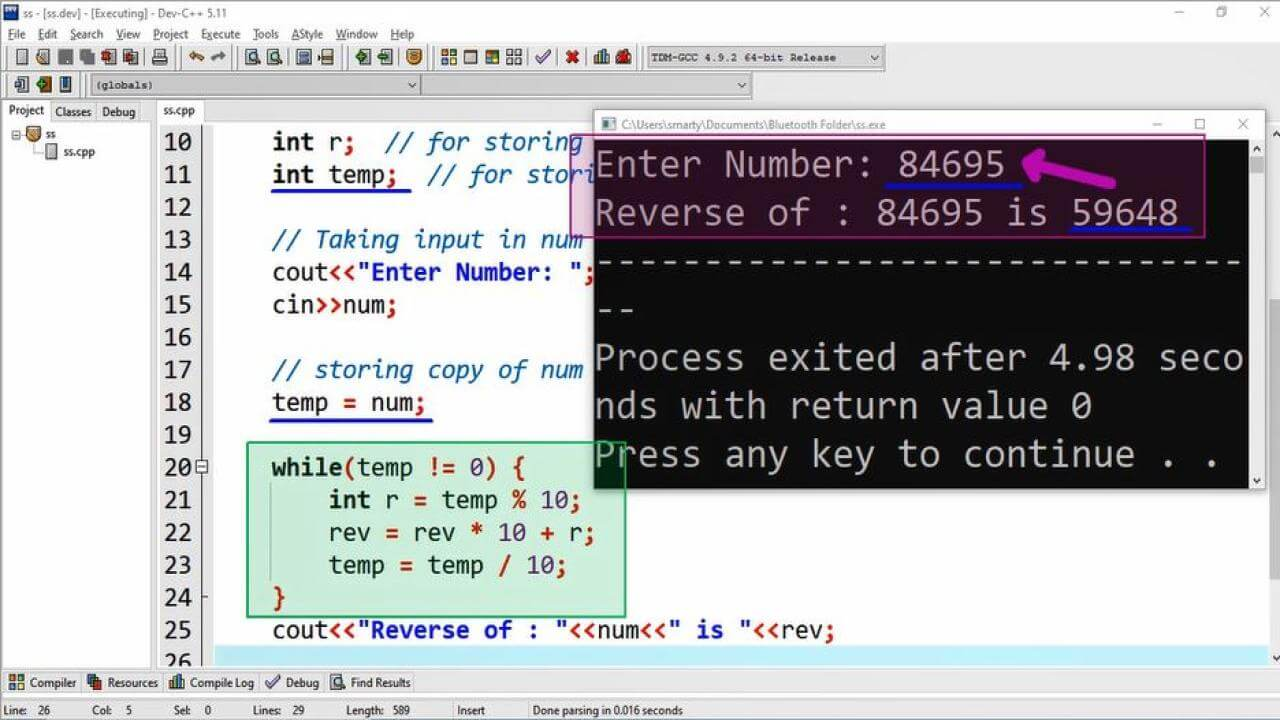# Reverse Number Using While Loop CPP program

## Source Code:

#include <iostream>
using namespace std;

## rev = 0;  // assigning 0 to rev variable.          // Taking input in num     cout<<"Enter Number: ";     cin>>num;          // storing copy of num in temp     temp = num;          while(temp != 0) {         r = temp % 10;         rev = rev * 10 + r;         temp = temp / 10;     }     cout<<"Reverse of : "<<num<<" is "<<rev;          return 0;} Output:## Working:

This progam implement the algorithm that how we can input a number from user and find its reverse. Means that if user input a number 3645 then the this program will reverse its digits in that way 5463 and print on screen. For this purpose first of all we have to declare the required variables for calculation of reverse number. Here is the detail of variables along with description and their data type.

 Sr. Identifier Data Type Description 1 num int This variable is used to store the input taken from user. 2 rev int This variable will be used to stroe calculated reverse value. 3 r int r variable is used in while loop to store remainder of given number in while loop. 4 temp int temp variable is used to store copy of orignal input taken in num.

After taking input in num variable. We have used while loop for reverse calculation. In while loop the following steps are repeated until the value of temporary variable equals to zero.

1. Take remainder of numbr by 10
2. Put remainder in formula of reverse number
3. Reduce the number by 10

In step 1 and step 3 we use 10 to take remainder and reduce the given number in while loop. The reason is that we are going to find the reverse of decimal number, and base of reverse number is 10. So that here we use 10.

Similarly in step 2 we reverse formula, where we have multiply rev variable by 10 and then multiply it with rmainder calculated in r variable. In forst iteration the rev will have 0 and the result of 10 * 0 will be 0 and after sum the first iteration will return last digit of number in rev variable.

Before while loop we save copy of input number in temp variable. The reason to store its copy is that we are using this temp variable in iteration for calculation. An in this iteration temp variable will goes to zero. This is optional means this is not part of reverse calculation.

## Iterative Stpes:

Here we take example where we consider that input value from user is 5784 (This value may be different by user)

 Iteration r rev temp First 4 4 578 Second 8 48 57 Third 7 487 5 Forth 5 4875 0